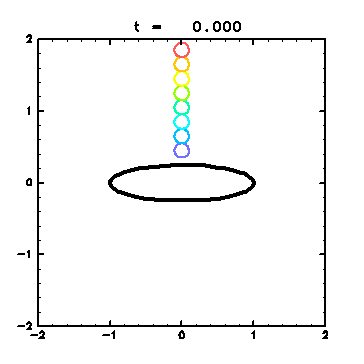4. Results
4.1 Time Evolution of an Unstable Elliptical Vortex
4.2 Analysis on the Streamfunction
4.3 Visualization with Passive Contours
4.4 Finite-Time Lyapunov Analysis on Fluid Deformation
4.5 Check by Passive Contour Advection

### 4.5 Check by Passive Contour AdvectionTo check the results of finite-time Lyapunov analysis in section 4.4 , passive contours are advected as in section 4.3 so that stretching of fluid particles can be understood intuitively. Initial conditions are given as follows: Vortex patch: same as in section 4.1 ( thick line ). Passive contour: eight circular contours with the radius 0.1 , centers of which are on x = 0, y = 0.45, 0.65,..., and 1.85 ( blue to red ). Animation 3: Advection of passive contours (GIF animation; 339kB). The contour positions are displayed from t = 0.0 to t = 10.0 at an interval of 0.1 . Thick line is the vortex patch. - As early as t = 4.0 , the yellow and green circles are stretched out, corresponding to the areas with large Lyapunov exponents in Fig. 4 . - Blue and light blue contours keep their shapes corresponding to the areas with small Lyapunov exponents. - In the co-rotating frame with the ellipse, fluids in these areas are rotating oppositely from the vortex, which visualizes the clockwise flow in Fig. 5 .

<<index <previous next>